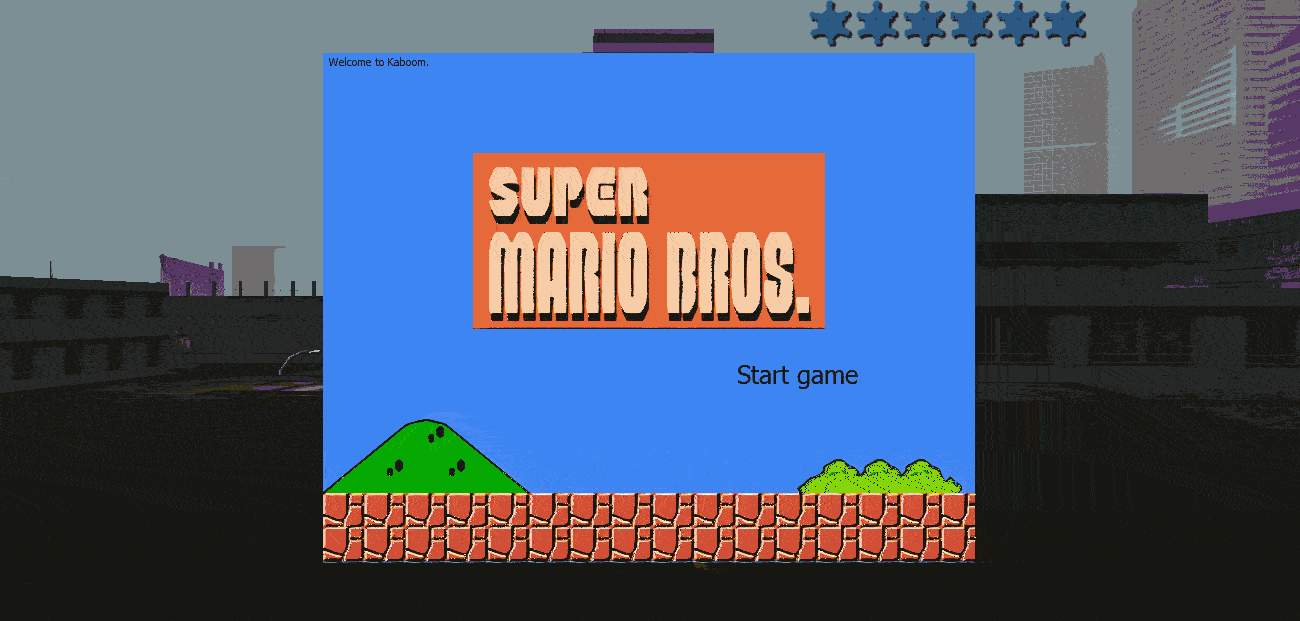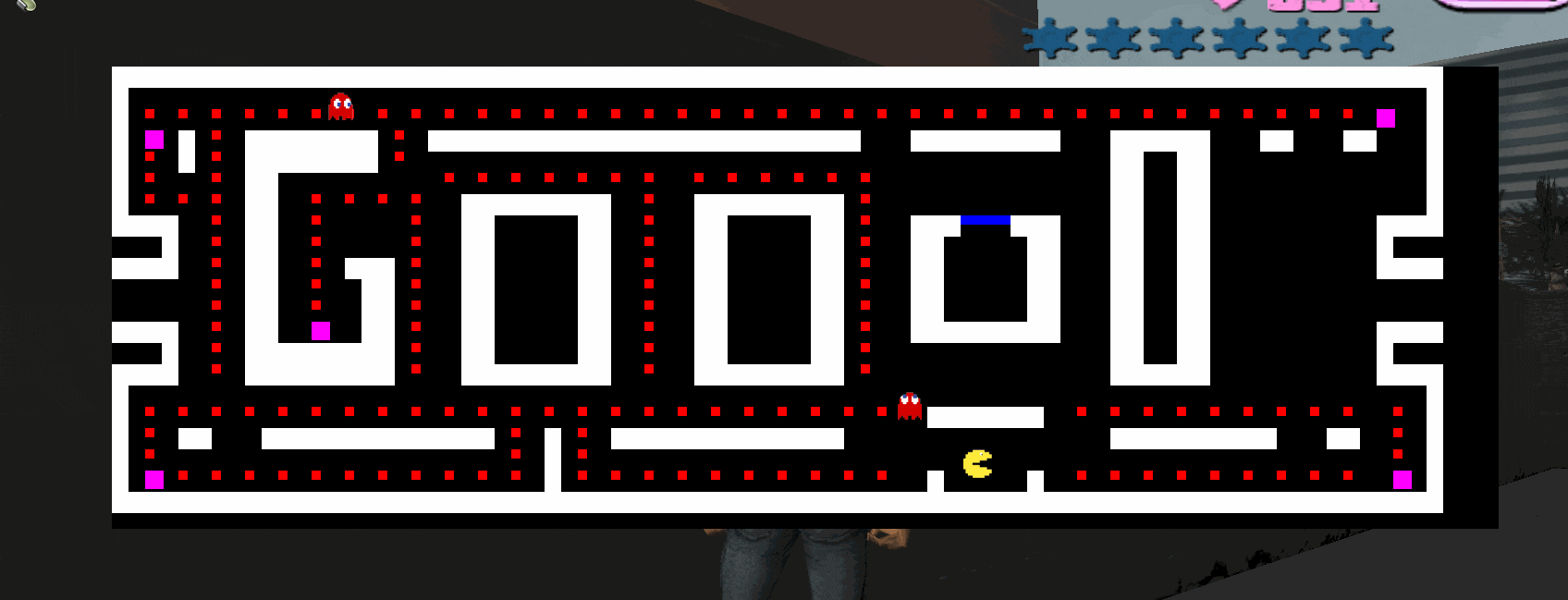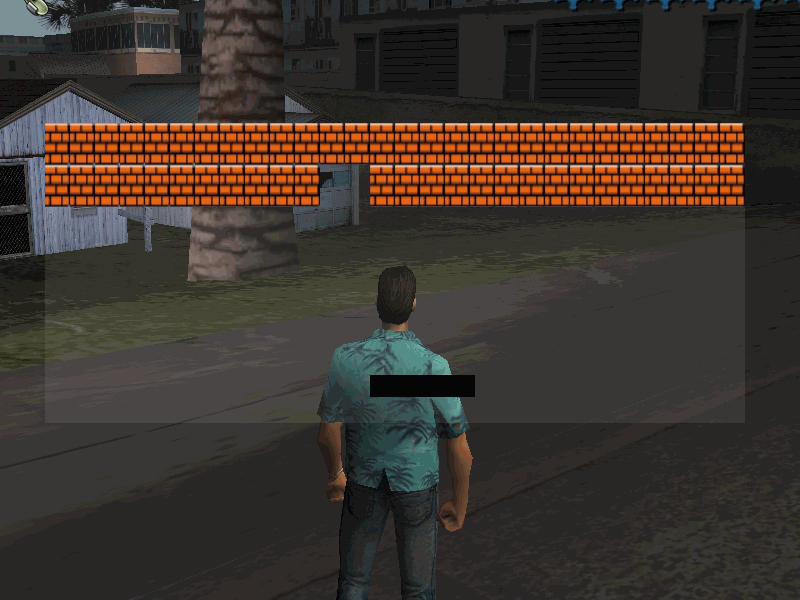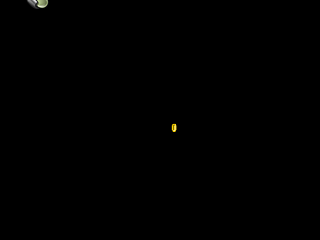#### AroliS^##### Kaboom - 2d Game Development.
«  »Last edited
based on Kaboom.jsKaboom.nut its a squirrel library that helps you make fast and easy 2d games.
For documentation and Installation guide check out Kaboom.nut github

Scene
Scenes are the different stages of a game, like different levels, menu screen, and start screen etc. Everything belongs to a scene.

Code: [Select]
`scene("level1", () => {     config ({      })});scene("level2", () => {     config ({      })});scene("gameover", () => {     config ({      })});start("level1"); // starting first level`

Mario

game code: https://github.com/AroliSG/Kaboom/blob/main/Examples/mario.nutPac-man

game code: https://github.com/AroliSG/Kaboom/blob/main/Examples/pacman.nutPlat-form

game code: https://github.com/AroliSG/Kaboom/blob/main/Examples/platform.nutTransform

game code: https://github.com/AroliSG/Kaboom/blob/main/Examples/transform.nutWarnings

• collision its not perfect with gravity games. 'Looking forward for future improvements'
• before using any example make sure, all sprites are located at sprite/kaboom/.
• this topic will be updated periodically, but stay tuned on github.
• when using gravity with objects. You shouldn't use pos.Y which will overpass the wall/objects body, you should use 'Jump ()'

Important: Library still under development, if you have found a bug. Feel free to create an issue on github.
Feel free to leave your feedback,

what games can we make with this library?
As far as your imagination can go.
If you would like to assist this project, follow the github and pull request any time.

I have made a file of sprites
Lemme love ya

#### We3da##### Re: Kaboom - 2d Game Development.
Great project and i think it will be useful in the next generation.
If you hide the vc screen it would be better. @AroliS^
After all this pain, peace will come.

#### Sebastian##### Re: Kaboom - 2d Game Development.
Woah! Nice!#### AroliS^##### Re: Kaboom - 2d Game Development.
Hi, Im here once more time!

Kaboom have been rewritten, which now is more stable and more efficienty.
spritesheet have been added, all credits goes to DMWarrior, which I just took his function and rewrite it to Kaboom.
now, It's possible to move among stages see the video - https://i.imgur.com/rbLjznx.mp4

Sample games
mario was the only game rewritten for this new version.

sprites list: https://imgur.com/a/z0y1Eie##### Re: Kaboom - 2d Game Development.
new game: Pac - man
Code: [Select]
`local Kaboom = Kab ({    id = "pacman"    loadRoot = "kaboom/"})  local obj = {    "|": {        square      = true        alias       = "blocks"        solid       = true    }    "-": {        square      = true        alias       = "blocks"        solid       = true    }    ".": {        square      = true        alias       = "points"        solid       = true        Size = VectorScreen (10,10)        Colour = Colour (255,0,0)    }         "_": {        square      = true        alias       = "door"        solid       = true        Size = VectorScreen (0,10)        Colour = Colour (0,0,255)    }      "@": {        square      = true        alias       = "bigpoints"        solid       = true        Size = VectorScreen (20,20)        Colour = Colour (255,0,255)    }        } local map = [    "|------------------------------------------------------------------------------|"    "|                                                                              |"    "| . . . . . . . . . . . . . . . . . . . . . . . . . . . . . . . . . . . . . @  |"    "| @ - . -------- . --------------------------   ---------   ------   --   --   |"    "| . - . -------- .                                          ||  ||             |"    "| .   . ||          . . . . . . .  . . . . . .              ||  ||             |"    "| . . . ||  . . . .  ---------  .  --------- .              ||  ||             |"    "|---  . ||  .     .  ||     ||  .  ||     || .  ---___---   ||  ||          ---|"    "   |  . ||  .     .  ||     ||  .  ||     || .  ||     ||   ||  ||          |   "    "----  . ||  . --- .  ||     ||  .  ||     || .  ||     ||   ||  ||          ----"    "      . ||  .  || .  ||     ||  .  ||     || .  ||     ||   ||  ||              "    "      . ||  .  || .  ||     ||  .  ||     || .  ||     ||   ||  ||              "    "----  . ||  @  || .  ||     ||  .  ||     || .  ---------   ||  ||          ----"    "   |  . ||-----|| .  ||     ||  .  ||     || .              ||  ||          |   "    "|---  . --------- .  ---------  .  --------- .              ------          ---|"    "|                                                                              |"    "| . . . . . . . . . . . . . . . . . . . . . . .  -------  . . . . . . . . .  . |"    "| . --   -------------- . | . --------------                ----------   --  . |"    "| .                     . | .                                                . |"    "| @ . . . . . . . . . . . | . . . . . . . . . .  |     |  . . . . . . . . .  @ |"    "|------------------------------------------------------------------------------|"] Kaboom.scene ("one", function () {    config ({        bgcolor = [0,0,0]        sceneBorder = true        Size = VectorScreen (1500,500)    });     addLevel (map, obj);    local up = array(5, null), down = array(5, null), left = array(5, null), right = array(5, null);    local getAnimPos = function (x, y) {        if (x == -5) return 0;        if (x == 5) return 1;        if (y == 5) return 2;        if (y == -5) return 3;    }      local getItWork = function (entity, Id) {        local pos = [{X = 5, Y = 0}, {X = -5, Y = 0}, {X = 0, Y = 5}, {X = 0, Y = -5}];        local random = function (start, finish) return ((rand() % (finish - start)) + start);                if (entity.props ().getBumpHeading () == "up") {            if (up [Id]) entity.move2d (up [Id].X, up [Id].Y);             else {                up [Id] = pos[random (0, 4)];                if (up [Id].Y == -5) up [Id] = null;            }            down  [Id]  = null;            left  [Id]  = null;            right [Id]  = null;                // animations            if (up [Id]) entity.playAnim ([getAnimPos (up [Id].X, up [Id].Y)]);        }        else if (entity.props ().getBumpHeading () == "down") {            if (down [Id]) entity.move2d (down [Id].X, down [Id].Y);             else {                 down [Id] = pos[random (0, 4)];                if (down [Id].Y == 5) down [Id] = null            }            up    [Id]  = null;            left  [Id]  = null;            right [Id]  = null;                // animations            if (down [Id]) entity.playAnim ([getAnimPos (down [Id].X, down [Id].Y)]);        }                else if (entity.props ().getBumpHeading () == "left") {            if (left [Id]) entity.move2d (left [Id].X, left [Id].Y);             else {                left [Id] = pos[random (0, 4)];                if (left [Id].X == -5) left [Id] = null;            }            up    [Id]  = null;            right [Id]  = null;            down  [Id]  = null;                  // animations            if (left [Id])  entity.playAnim ([getAnimPos (left [Id].X, left [Id].Y)]);        }                else {            if (right [Id]) entity.move2d (right [Id].X, right [Id].Y);             else {                right [Id]= pos[random (0, 4)];                if (right [Id].X == 5) right [Id]= null            }            up   [Id]  = null;            left [Id]  = null;            down [Id]  = null;                   // animations            if (right [Id]) entity.playAnim ([getAnimPos (right [Id].X, right [Id].Y)]);           }    }    local enemy = addEntity ({        uniqueId    = "enemy1"        spriteId    = "pacsheet"        Pos         = getPos (18,30)        Size = VectorScreen (35,35)        spritesheet = {              Id  = 0, // will reproduce sprite id 5 in sprite sheet            UV  = VectorScreen (64, 64),             X   = 0.25,             Y   = 0.5        }        solid   = true        alias = "enemy"        Ignore = [ "points", "bigpoints" ]    })    local enemy2 = addEntity ({        uniqueId    = "enemy2"        spriteId    = "pacsheet"        Pos         = getPos (10,30)        Size = VectorScreen (35,35)        spritesheet = {              Id  = 0, // will reproduce sprite id 5 in sprite sheet            UV  = VectorScreen (64, 64),             X   = 0.25,             Y   = 0.5        }        solid   = true        alias = "enemy"        Ignore = [ "points", "bigpoints" ]    })        local Index = 0;    enemy.action (function () {         if (entity.collect ().has ("coloured"))  {            enemy.Colour = Colour (rand () * 255, rand () * 255, rand () * 255 );            enemy2.Colour = Colour (rand () * 255, rand () * 255, rand () * 255 );                        game.Timeout (function () {                    // enemy 1                enemy.collect ().remove ("coloured");                enemy.Colour = Colour (255,255,255); // returning original colour                     // enemy 2                enemy2.collect ().remove ("coloured");                enemy2.Colour = Colour (255,255,255); // returning original colour             }, 100)        }          getItWork (entity, 0);      })    enemy2.action (function () { getItWork (entity, 1); })    local pac = addEntity ({        uniqueId    = "pac"        spriteId    = "pacsheet"        Pos         = getPos (18,51)        Size        = VectorScreen (35,35)        spritesheet = {              Id  = 5, // will reproduce sprite id 5 in sprite sheet            UV  = VectorScreen (64, 64),             X   = 0.25,             Y   = 0.5        }        solid   = true        player  = true    })    pac.action (function () {        Key ("right", function () {            if (event == "down") {                entity.move2d (5, 0);                entity.playAnim ([6,5,4], {                    animSpeed = 0.5                })            }        })        Key ("left", function () {            if (event == "down") {                entity.move2d (-5, 0);                entity.playAnim ([6,5,4], {                    animSpeed = 0.5                    mirror = true                })            }        })        Key ("up", function () {            if (event == "down") {                entity.move2d (0, -5);                entity.playAnim ([6,5,4], {                    animSpeed = 0.5                    rotate = "up"                })            }        })        Key ("down", function () {            if (event == "down") {                entity.move2d (0, 5);                entity.playAnim ([6,5,4], {                    animSpeed = 0.5                    rotate = "down"                })             }        })            })    pac.collides ("points", function () {        hit.entity.destroy2d ();    })    pac.collides ("bigpoints", function () {        hit.entity.destroy2d ();        // add the 'data' coloured to the collection of the entity for an enemy effect.        enemy.collect ().set ("coloured", true);        enemy2.collect ().set ("coloured", true);    })    pac.collides ("enemy", function () {        local obj = hit.entity;        if (obj.collect ().has ("coloured")) {            obj.hide ();        }        else {            entity.destroy2d ();        }    })    render (function () {         pac.render ();        enemy.render ();        enemy2.render ();    })})Kaboom.start ("one");`spritesheet: https://i.imgur.com/RdV1iXP.png

as all game done by me, aren't finished yet. so, keep tuned for the updates of these games and the Kaboom class.
Lemme love ya

#### Sebastian##### Re: Kaboom - 2d Game Development.